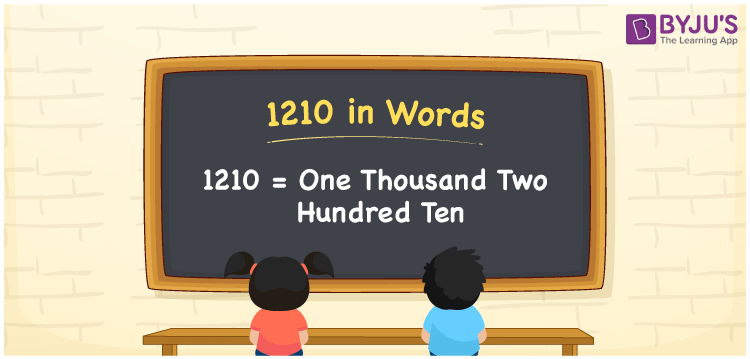# 1210 in Words

1210 in words is written as One thousand two hundred ten. In both the International System of Numerals and the Indian System of Numerals, 1210 is written as One thousand forty. The number 1210 is a Cardinal Number as it represents some quantity. For example, “that item costs 1210 rupees”.

 1210 in Words One thousand two hundred ten One thousand two hundred ten in Number 1210

## 1210 in English Words

1210 in English words is read as “One thousand two hundred ten”.## How to Write 1210 in Words?

To write 1210 in words, we shall use the place value chart. In the place value chart, put 1 in the thousands, 2 in the hundreds, 1 in the tens, and 0 in the ones, respectively. Let us make a place value chart to write the number 1210 in words.

 Thousands Hundreds Tens Ones 1 2 1 0

Thus, we can write the expanded form as

1 × Thousand + 2 × Hundred + 1 × Ten + 0 × One

= 1 × 1000 + 2 × 100 + 1 × 10 + 0 × 1

= 1000 + 200 + 10 + 0

= 1210

= One thousand two hundred ten.

1210 is a natural number which is the successor of 1209 and the predecessor of 1211.

1210 in words – One thousand two hundred ten

• Is 1210 an odd number? – No
• Is 1210 an even number? – Yes
• Is 1210 a perfect square number? – No
• Is 1210 a perfect cube number? – No
• Is 1210 a prime number? – No
• Is 1210 a composite number? – Yes

## Frequently Asked Questions on 1210 in Words

Q1

### How to write 1210 in words?

1210 in words is written as One thousand two hundred ten.
Q2

### How to write 1210 in words in the International and Indian System of Numerals?

In both, the system of numerals, 1210 in words, is written as One thousand two hundred ten.
Q3

### How to write 1210 in a place value chart?

In the place value chart, write 1 in the thousands, 2 in the hundreds, 1 in the tens, and 0 in the ones, respectively.